# But WHY is the Lattices Bounded Distance Decoding Problem difficult?

This is taken from my Master Thesis on Homomorphic Signatures over Lattices.

### Introduction to lattices and the Bounded Distance Decoding Problem

A lattice is a discrete subgroup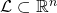, where the word discrete means that each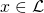has a neighborhood inthat, when intersected with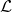results in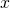itself only. One can think of lattices as being grids, although the coordinates of the points need not be integer. Indeed, all lattices are isomorphic to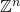, but it may be a grid of points with non-integer coordinates.Another very nice way to define a lattice is: given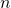independent vectors, the latticegenerated by that base is the set of all linear combinations of them with integer coefficients: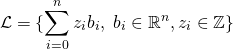Then, we can go on to define the Bounded Distance Decoding problem (BDD), which is used in lattice-based cryptography (more specifically, for example in trapdoor homomorphic encryption) and believed to be hard in general.

Given an arbitrary basis of a lattice, and a point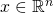not necessarily belonging to, find the point ofthat is closest to. We are also guaranteed thatis very close to one of the lattice points. Notice how we are relying on an arbitrary basis – if we claim to be able to solve the problem, we should be able to do so with any basis.

Now, as the literature goes, this is a problem that is hard in general, but easy if the basis is nice enough. So, for example for encryption, the idea is that we can encode our secret message as a lattice point, and then add to it some small noise (i.e. a small element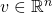). This basically generates an instance of the BDD problem, and then the decoding can only be done by someone who holds the good basis for the lattice, while those having a bad basis are going to have a hard time decrypting the ciphertext.

However, albeit of course there is no proof of this (it is a problem believed to be hard), I wanted to get at least some clue on why it should be easy with a nice basis and hard with a bad one (GGH is an example schema that employs techniques based on this).

So now to our real question. But WHY is the Bounded Distance Decoding problem hard (or easy)?

### Why the Bounded Distance Decoding problem is easy with a nice basis

Let’s first say what a good basis is. A basis is good if it is made of nearly orthogonal short vectors. This is a pretty vague definition, so let’s make it a bit more specific (although tighter): we want a base in which each of its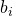is of the form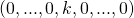for some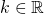. One can imagine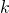being smaller than some random value, like 10. (This shortness is pretty vague and its role will be clearer later.) In other words, a nice basis is the canonical one, in which each vector has been re-scaled by an independent real factor.

To get a flavor of why the Bounded Distance Decoding problem is easy with a nice basis, let’s make an example. Consider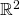, with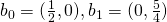as basis vectors. Suppose we are given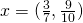as challenge point. It does not belong to the lattice generated by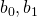, but it is onlyaway from the point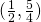, which does belong to the lattice.

Now, what does one have to do to solve this problem? Let’s get a graphical feeling for it and formalize it.

We are looking for the lattice point closest to. So, sitting on, we are looking for the linear combination with integer coefficients of the basis vectors that is closest to us. Breaking it component-wise, we are looking forand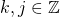such that they are solution of: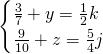This may seem a difficult optimization problem, but in truth it is very simple! The reason is that each of the equations is independent, so we can solve them one by one – the individual minimum problems are easy and can be solved quickly. (One could also put boundaries on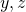with respect to the norm of the basis vectors, but it is not vital now.)

So the overall complexity of solving BDD with a good basis is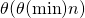, which is okay.

### Why the Bounded Distance Decoding problem is hard with a bad basis

A bad basis is any basis that does not satisfy any of the two conditions of a nice basis: it may be poorly orthogonal, or may be made of long vectors. We will later try to understand what roles these differences play in solving the problem: for now, let’s just consider an example again.

Another basis for the lattice generated by the nice basis we picked before (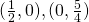) is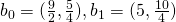. This is a bad one.

Let’s write down the system of equations coordinate-wise as we did for the nice basis. We are looking forandsuch that they are solution of: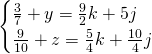Now look! This may look similar as before, but this time it really is a system, the equations are no longer independent: we have 3 unknowns and 2 equations. The system is under-determined! This already means that, in principle, there are infinite solutions. Moreover, we are also trying to find a solution that is constrained to be minimum. Especially with big, solving this optimization problem can definitely be non-trivial!

### On the differences between a good and a bad basis

So far so good: we have discovered why the Bounded Distance Decoding problem is easy with a good basis and difficult with a bad one. But still, what does a good basis have to make it easy? How do its properties related to easy of solution?

We enforced two conditions: orthogonality and shortness. Actually, we even required something stronger than orthogonality: that the good basis was basically a stretched version of the canonical one – i.e. had only one non-zero entry.

Let’s think for a second in terms of canonical basis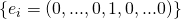. This is what makes the minimum problems independent and allows for easy resolution of the BDD problem. However, when dealing with cryptography matters, we cannot always use the same basis, we need some randomness. That is why we required to use a set of independent vectors each having only one non-zero coordinate: it is the main feature that makes the problem easy (at least for the party having the good basis).

We also asked for shortness. This does not give immediate advantage to who holds the good basis, but makes it harder to solve the problem for those holding the bad one. The idea is that, given a challenge point, if we have short basis vectors, we can take small steps from it and look around us for nearby points. It may take some time to find the best one, but we are still not looking totally astray. Instead, if we have long vectors, every time we use one we have to make a big leap in one direction. In other words, who has the good basis knows the step size of the lattice, and thus can take steps of considerate size. slowly poking around; who has the bad basis takes huge jumps and may have a hard time pinpointing the right point.

It is true, though, that the features of a good basis usually only include shortness and orthogonality, and not the “rescaling of the canonical basis” we assumed in the first place. So, let’s consider a basis of that kind, like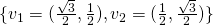. If we wrote down the minimum problem we would have to solve given a challenge point, it would be pretty similar to the one with the bad basis, with the equations not being independent. Looks like bad luck, uh?

However, not all hope is lost! In fact, we can look for the rotation matrix that will turn that basis into a stretching of the canonical one, finding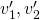! Then we can rotate the challenge pointas well, and solve the problem with respect to those new basis vectors. Of course that is not going to be the solution to the problem, but we can easily rotate it back to find the real solution!

However, given that using a basis of this kind does not make the opponent job any harder, but only increases the computational cost for the honest party, I do not see why this should ever be used. Instead, I guess the best choices for good basis are the stretched canonical ones.

(This may be obvious, but having a generic orthogonal basis is not enough for an opponent to break the problem. If it is orthogonal, but its vectors are long, bad luck!)